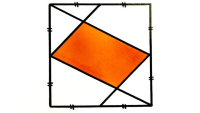# Catriona Agg’s Puzzles from 2020

1. four triangles in a square Dec 31, 2020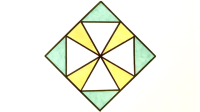2. zig-zag inside a rectangle Dec 30, 2020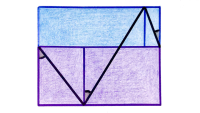3. five squares across a square Dec 23, 2020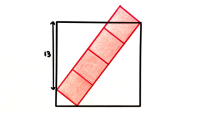4. two squares and a semi-circle Dec 13, 2020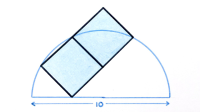5. two circles in a square ii Oct 31, 2020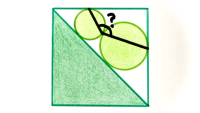6. triangles formed from rectangles Oct 29, 2020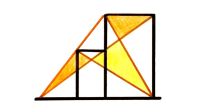7. three triangles in a circle in a triangle Oct 24, 2020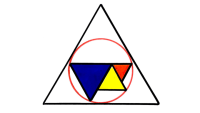8. two quarter circles in a semi-circle Aug 31, 2020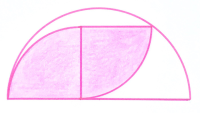9. circle inside a triangle Aug 28, 2020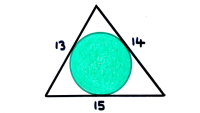10. similar rectangles Aug 26, 2020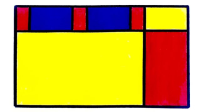11. two semi-circles in a circle Aug 21, 2020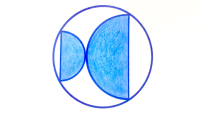12. semi-circle and quarter circle in a square Aug 17, 2020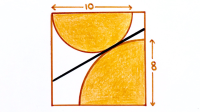13. multiple semi-circles in a circle Aug 15, 2020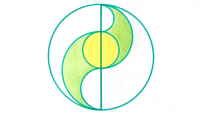14. four squares iii Aug 14, 2020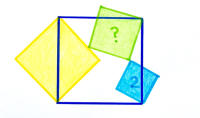15. triangle over two rectangles Aug 7, 2020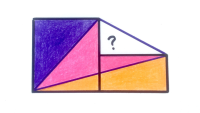16. subdivided decagon Jul 30, 2020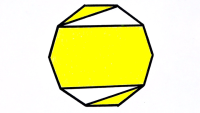17. two congruent rectangles and a semi-circle Jul 29, 2020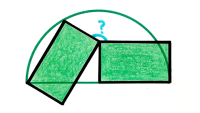18. a rectangle in a square Jul 26, 2020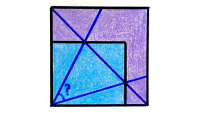19. two half-squares and a circle Jul 25, 2020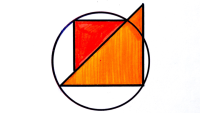20. three congruent rectangles ii Jul 23, 2020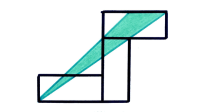21. semi-circle in a square in a quarter circle Jul 19, 2020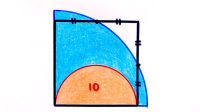22. two triangles and three concentric semi-circles Jul 18, 2020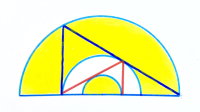23. five congruent rectangles Jul 15, 2020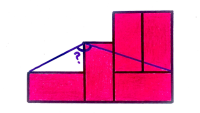24. five rectangles tiling a square Jul 11, 2020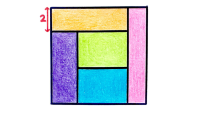25. rectangle overlapping a circle Jul 5, 2020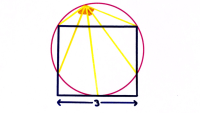26. swirling semi-circles Jun 14, 2020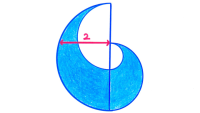27. hexagon in a quarter circle May 31, 2020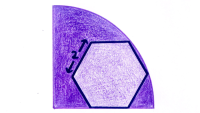28. six semi-circles inside a hexagon May 30, 2020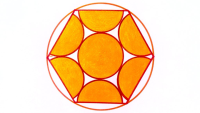29. four quarter circles May 29, 2020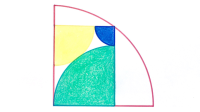30. two overlapping triangles and a circle May 28, 2020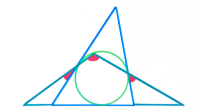31. two squares diagonally inside a rectangle May 26, 2020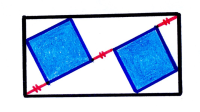32. two circles and two semi-circles May 25, 2020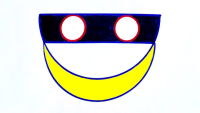33. two tilted squares May 23, 2020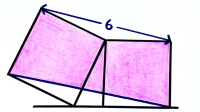34. a circle and two semi-circles in two hexagons May 14, 2020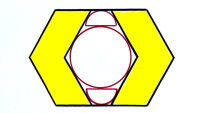35. four squares overlapping a rectangle May 9, 2020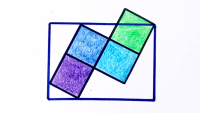36. two semi-circles and a triangle May 8, 2020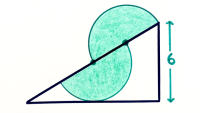37. a square and a hexagon May 5, 2020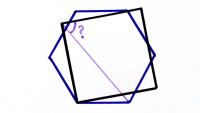38. five circles in a square May 2, 2020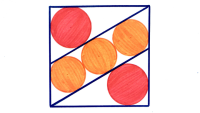39.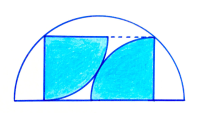40. four squares ii Apr 28, 2020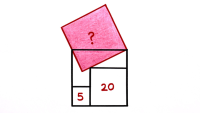41. four squares Apr 26, 2020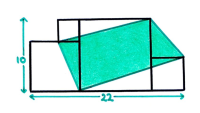42. two circles inside an equilateral triangle Apr 25, 2020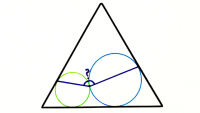43. three congruent rectangles Apr 24, 2020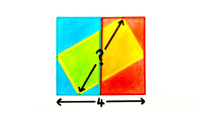44. four congruent squares inside a larger square Apr 23, 2020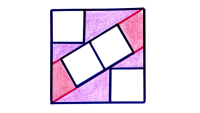45. two semi-circles and an equilateral triangle Apr 22, 2020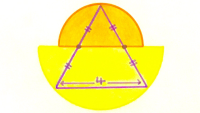46. two quarter circles and a semi-circle Apr 21, 2020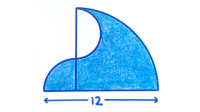47. three stacked squares Apr 19, 2020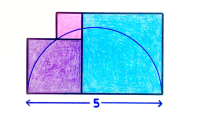48. two circles in a square Apr 18, 2020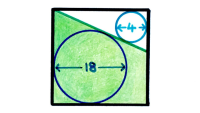49. three overlapping rectangles ii Apr 17, 2020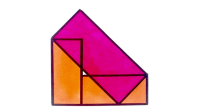50. semi-circle splitting a triangle Apr 16, 2020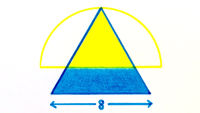51. two semi-circles diagonally across a square Apr 15, 2020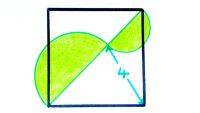52. two neighbouring rectangles Apr 13, 2020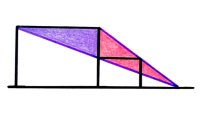53. two overlapping semi-circles Apr 12, 2020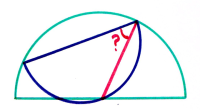54. nested semi-circles Apr 11, 2020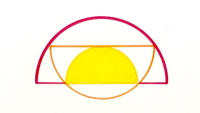55. two circles in a triangle Apr 10, 2020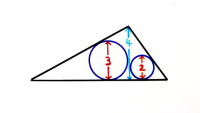56. tangential hexagon and circle Apr 9, 2020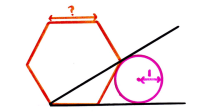57. three overlapping rectangles Apr 8, 2020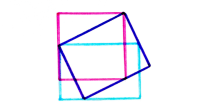58. three circles forming a triangle Apr 7, 2020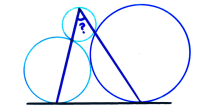59. four squares in a circle Apr 6, 2020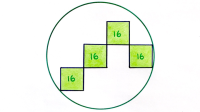60. three equal area rectangles Apr 5, 2020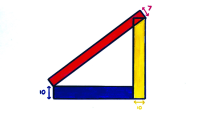61. six equal area rectangles Apr 4, 2020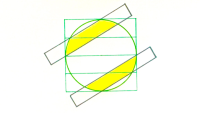62. angle formed by three squares Apr 2, 2020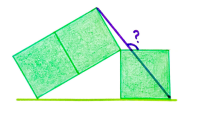63. quadrilateral splitting a semi-circle Apr 1, 2020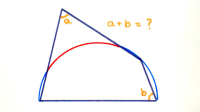64. two regular hexagons Mar 28, 2020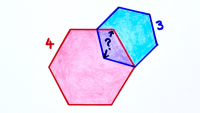65. three squares in a circle Mar 21, 2020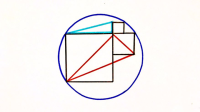66. two squares and an equilateral triangle Mar 14, 2020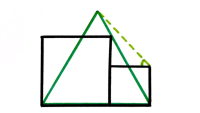67. quarter circles in a rectangle in a circle Mar 7, 2020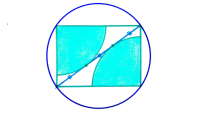68. nested isosceles triangles Mar 5, 2020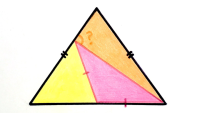69. five circles in a rectangle in a semi-circle Feb 29, 2020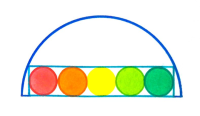70. two semi-circles inside a rectangle Feb 23, 2020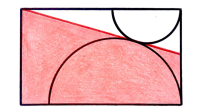71. two squares inside an equilateral triangle Feb 22, 2020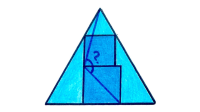72. tilted rectangle in a square Feb 20, 2020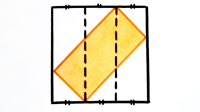73. circle in a quarter circle Feb 18, 2020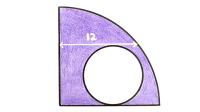74. three semi-circles Feb 16, 2020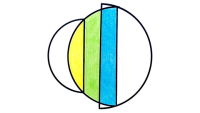75. a square and a rectangle overlapping Feb 14, 2020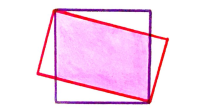76. three circles in a hexagon in a circle Feb 10, 2020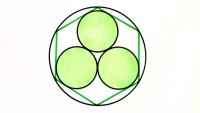77. three circles Feb 8, 2020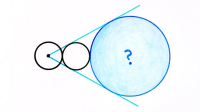78. three squares in a triangle Feb 4, 2020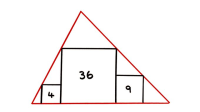79. four squares Feb 1, 202080. arcs in a semi-circle Jan 30, 2020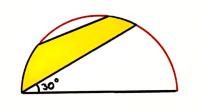81. three isoscelese triangles inside a triangle Jan 23, 2020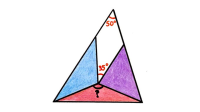82.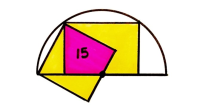83. three stacked squares and a fourth square Jan 12, 2020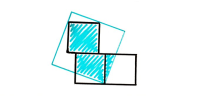84. parallelogram area Jan 7, 2020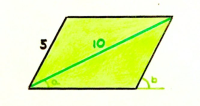85. three squares Jan 4, 2020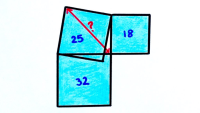86. parallelogram in a rectangle Jan 1, 2020